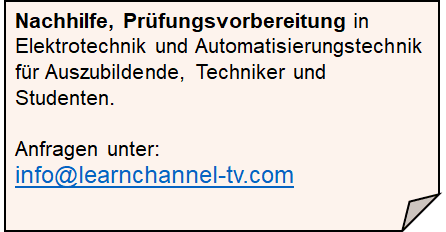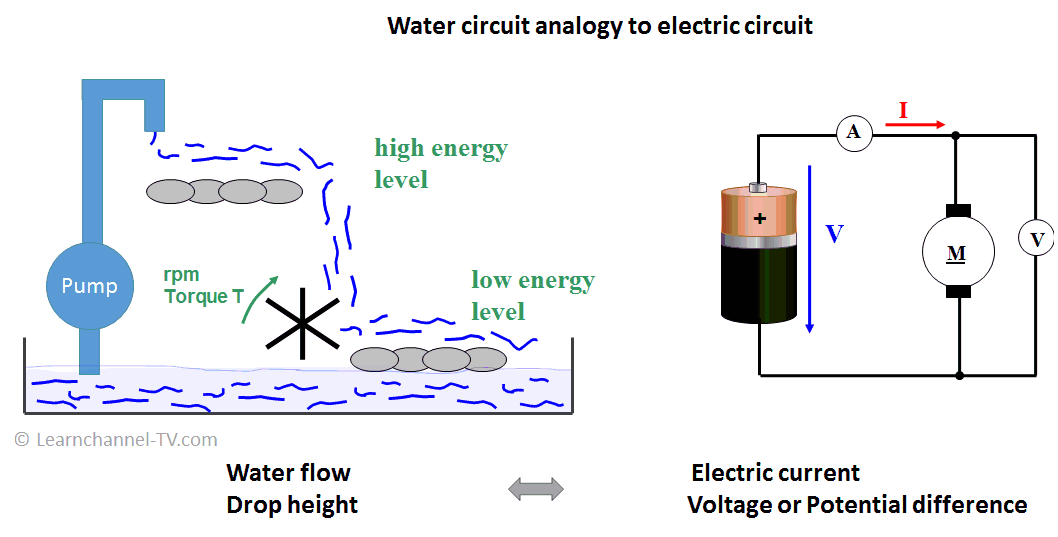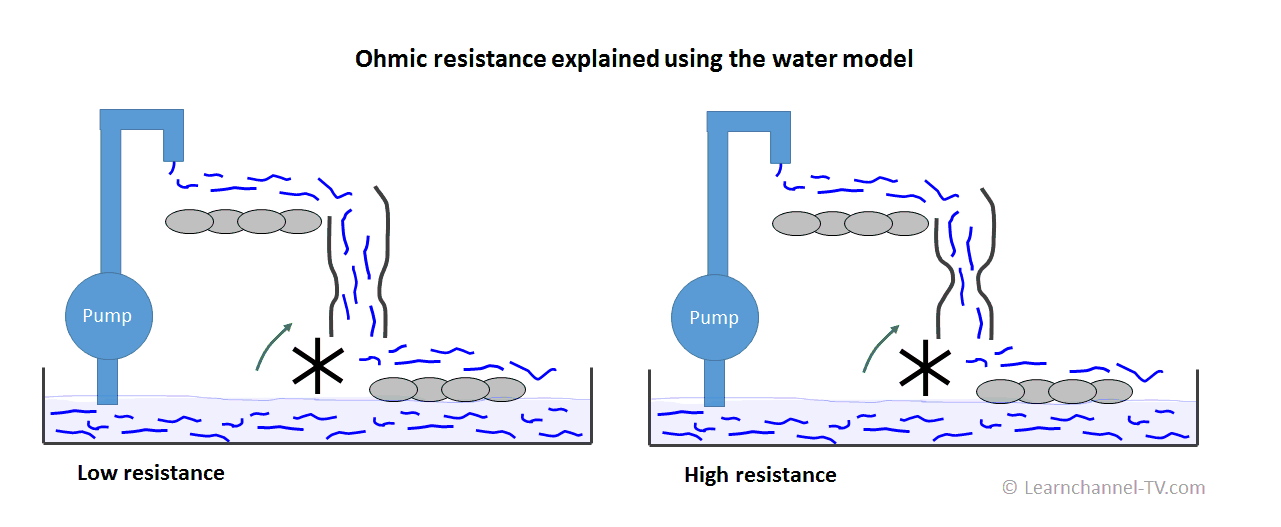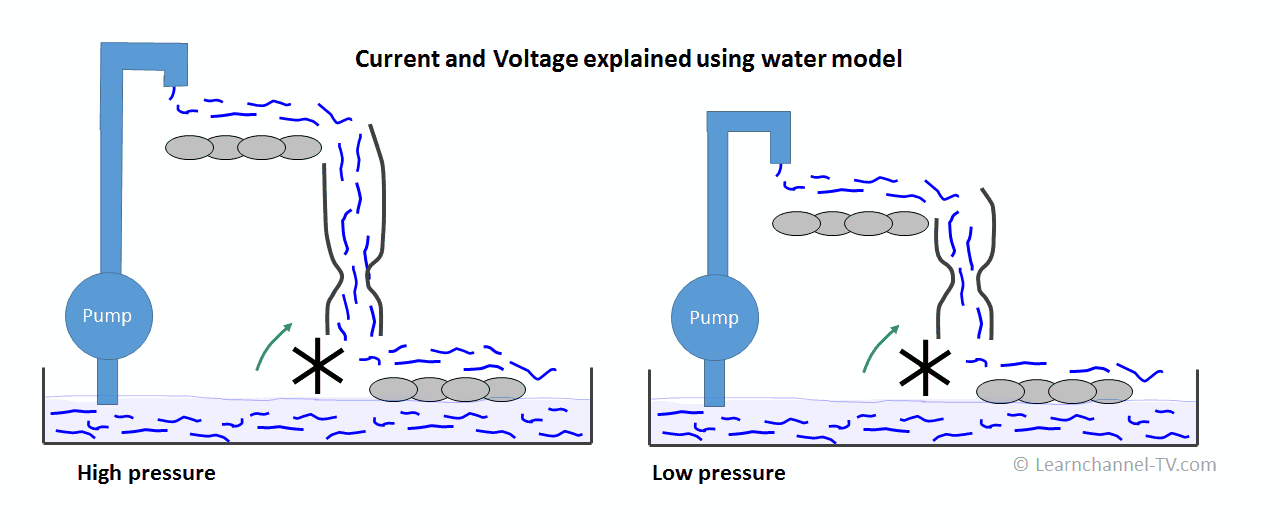(Deutsch)Learnchannel Nachhilfe

## How to explain what is electric current, voltage and resistance?

How can the terms "current", "voltage", "resistance" and "electrical power" be explained without having to go too deep into physics? One possibility would be the water model:Water circuit analogy to electric circuit

We compare:

Water flow or amount of water     <=>     Electrical current I (unit A Ampere)

Fall height water                              <=>     Voltage V (unit V volt)

How can the Electrical power P be derived? Also using a water model:

When does the water wheel turn the faster? The larger the quantity of water.  =>   P ∼ I

When is the torque of the waterwheel the greater?  The greater the fall height of the water.    =>   P ∼ U

=>      P = U * I       Unit: W Watt

### Ohm's law is explained using the water model

Ohm's law describes the relationship between voltage, current and resistance. Here too, one can get an explanation with the help of the water model:Ohmic resistance explained by using the water model

You can see: When there is great resistance, we have a small (water) current and the water wheel turns slowly.

I ∼ 1 ⁄ R    ...(1)Voltage and Current explained using water model

As you see: The greater the height of fall or pressure of the water, the greater the water flow and the faster the water wheel turns. According to our analogy the following proportionality applies:  I ∼ V   ...(2)

If you combine both proportions ... (1) and ... (2), you get the so-called  Law of Ohm:

I =  V ⁄ R   or  R = V ⁄ I   or V = R * I

R stands for Ohmic resistor in Ω (Ohm)